Courses

# Practice Test: Pair of Linear Equations in Two Variables

## 25 Questions MCQ Test Mathematics (Maths) Class 10 | Practice Test: Pair of Linear Equations in Two Variables

Description
This mock test of Practice Test: Pair of Linear Equations in Two Variables for Class 10 helps you for every Class 10 entrance exam. This contains 25 Multiple Choice Questions for Class 10 Practice Test: Pair of Linear Equations in Two Variables (mcq) to study with solutions a complete question bank. The solved questions answers in this Practice Test: Pair of Linear Equations in Two Variables quiz give you a good mix of easy questions and tough questions. Class 10 students definitely take this Practice Test: Pair of Linear Equations in Two Variables exercise for a better result in the exam. You can find other Practice Test: Pair of Linear Equations in Two Variables extra questions, long questions & short questions for Class 10 on EduRev as well by searching above.
QUESTION: 1

### If A : Homogeneous system of linear equations is always consistent. R : x = 0, y = 0 is always a solution of the homogeneous system of equations with unknowns x and y, then which of the following statement is true?

Solution:

Homogeneous system of  linear equations are of the form ax+by=0 and cx+dy=0. These lines pass through the origin. Thus, there is always at least one solution, the point (0,0).So, Homogeneous system of linear is always consistent, meaning that it has at least one solution which is (0,0). So B is the exact explanation.

QUESTION: 2

Solution:
QUESTION: 3

### The pair of linear equations 2x + ky – 3 = 0, 6x + 2/3y + 7 = 0 has a unique solution if –

Solution:
QUESTION: 4

The pair of linear equations 2kx + 5y = 7, 6x – 5y = 11 has a unique solution if –

Solution:
QUESTION: 5

The pair of equations 3x + 4y = k, 9x + 12y = 6 has infinitely many solutions if –

Solution:

An equation has infinitely many solutions when the lines are coincident.
The lines are coincident when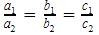So 3x + 4y = k, 9x + 12y = 6 are coincident when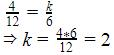QUESTION: 6

The pair of linear equations 2x + 5y = k, kx + 15y = 18 has infinitely many solutions if –

Solution:

An equation has infinitely many solutions when the lines are coincident.
The lines are coincident when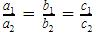So 2x + 5y = k, kx + 15y = 18 are coincident when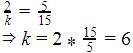QUESTION: 7

The pair of linear equations 3x + 5y = 3, 6x + ky = 8 do not have any solution if –

Solution: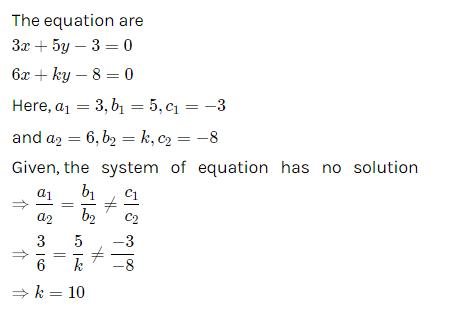QUESTION: 8

The pair of linear equations 3x + 7y = k, 12x + 2ky = 4k + 1 do not have any solution if

Solution:
QUESTION: 9

8 girls and 12 boys can finish work in 10 days while 6 girls and 8 boys can finish it in 14 days. Find the time taken by the one girl alone that by one boy alone to finish the work.

Solution:
QUESTION: 10

The pair of linear equations kx + 4y = 5, 3x + 2y = 5 is consistent only when –

Solution:

kx + 4y = 5, 3x + 2y = 5
Here, a1=k,b1=4,c1​=−5
and a2=3,b2=2,c2=-5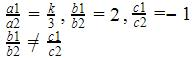So , The equation is consistent when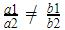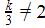k ≠ 6

QUESTION: 11

The pair of linear equations 7x + ky = k, 14x + 2y = k + 1 has infinitely many solutions if –

Solution:
QUESTION: 12

The pair of linear equations 13x + ky = k, 39x + 6y = k + 4 has infinitely many solutions if –

Solution:
QUESTION: 13

The pair of linear equations x + y = 3, 2x + 5y = 12 has a unique solution x = x1, y = y1 then value of x1 is –

Solution:
QUESTION: 14

The pair of linear equations 3x – 5y + 1 = 0, 2x – y + 3 = 0 has a unique solution x = x1, y = y1 then y1 =

Solution:
QUESTION: 15

The pair of linear equations x + 2y = 5, 7x + 3y = 13 has a unique solution -

Solution:
QUESTION: 16

The pair of linear equations x + 2y = 5, 3x + 12y = 10 has –

Solution:
QUESTION: 17

If the sum of the ages of a father and his son in years is 65 and twice the difference of their ages in years is 50, then the age of the father is –

Solution:
QUESTION: 18

A fraction becomes  4/5 when 1 is added to each of the numerator and denominator. However, if we subtract 5 from each then it becomes 1/2. The fraction is –

Solution:
QUESTION: 19

Three chairs and two tables cost Rs. 1850. Five chairs and three tables cost Rs. 2850. Then the total cost of one chair and one table is –

Solution:
QUESTION: 20

Six years hence a man's age will be three times the age of his son and three years ago he was nine times as old as his son. The present age of the man is –

Solution:

Let the present age of man is x and of son is y.
Six years hence,
Man’s age =x+6
Son’s age=y+6
Man’s age is 3 times son’s age
x+6=3(y+6)
x+6=3y+18
x=3y+12    …...1
Three years ago,
Man’s age =x-3
Son’s age=y-3
Man’s age was 9 times as of son
x-3=9(y-3)
x-3=9y-27
x=9y-24   ….2
From 1 and 2
3y+12=9y-24
6y=36
y=6
x=3*6+12=18+12=30 years

QUESTION: 21

Solve : 3x – 7 > x + 1, x ∈ R :

Solution:

3x - 7 > x + 1, x ∈ R
3x - x > 1 + 7
2x > 8
x > 4
x ∈ (4,∞)

QUESTION: 22

Solve : x/5R : <3x-2/4-5x-3/5, x ∈ R:

Solution:

Solution :

The correct option is Option C.

x/5 < (3x - 2)/4 - (5x - 3)/5

Now multiply throughout with 20,

4x < 5(3x - 2) - 4(5x - 3)

4x < 15x - 10 - 20x + 12

20x - 15x + 4x < 12 - 10

9x < 2

x < 2/9

So, x can be any real number in the interval (- infinity, 2/9)

QUESTION: 23

Solve : 2(x-1)/5<3(2+x)/7, x ∈ R :

Solution: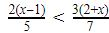7(2x-2)
<5(6+3x)
14x-14<30+15x
15x-14x<-30-14
x< -44
x ∈ (-∞,-44)

QUESTION: 24

Solve : 5x/2+3x/4>39/4, x ∈ R :

Solution:
QUESTION: 25

Solve : x-1/3+4 < x-5/5-2, x ∈ R

Solution: